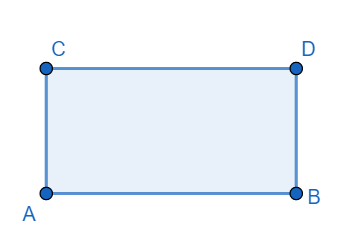QuestionAnswers

# The length of a rectangular park is 0.140km, and the breadth is 80m. What is the perimeter of the park? Three rounds of barbed wire are needed to fence the park. Find the cost of fencing the park at a rate of Rs 2.20 per metre.Hint: Use the fact that the perimeter of a rectangle = 2(l+b) where l is the length of the rectangle and b is the breadth of the rectangle. Hence find the total length of barbed wire required to fence the rectangle three rounds with the barbed wire. Hence find the cost of fencing at the rate of Rs.2.20 per metre.We have the length of the rectangle = l = AB = 0.140 km
Since 1km = 1000m, we have
AB = l = 140m
Also the breadth of the rectangle = AC = 80m.
Now, we have the perimeter of the rectangle = AB+AC+BD+CD
Since opposite sides of a parallelogram are equal, we have
AB = CD, AC = BD.
Hence the perimeter of the rectangle = l+b+l+b = 2l+2b.
Now l = 140 m and b = 80m
Hence the perimeter of the rectangle $=2\times 140+2\times 80=280+160=440m$
Hence the perimeter of the rectangle = 440m.
The length of the barbed wire to fence the rectangle three rounds $=440\times 3=1320m$
Hence the cost of fencing @Rs. 2.20/m $=1320\times 2.20=2904$
Hence the cost of fencing = Rs 2904

Note: In these types of questions take special care about the units of each of the given dimensions. It is very important to know the basic conversion of the units and making the units the same when calculating. Many students forget to do so and hence get incorrect results.
The Difference Between an Animal that is A Regulator and One that is A ConformerRelation Between the Length of a Given Wire and Tension for Constant Frequency Using SonometerWhat is the full form of phd?Where There is a Will There is a Way EssayThe Making of a ScientistChanging the Period of a PendulumWhat is the Difference Between Trade and Commerce?Group Theory in MathematicsWhat is the Cell Envelope?What is the Placebo EffectImportant Questions for CBSE Class 6 English A Pact with The Sun Chapter 9 - What Happened to The ReptilesImportant Questions for CBSE Class 6 English A Pact with The Sun Chapter 6 - The Monkey and the CrocodileImportant Questions for CBSE Class 6 English A Pact with The Sun Chapter 8 - A Pact with the SunImportant Questions for CBSE Class 9 Maths Chapter 9 - Areas of Parallelograms and TrianglesImportant Questions for CBSE Class 9 Science Chapter 4 - Structure of The AtomImportant Questions for CBSE Class 7 English An Alien Hand Chapter 9 - A Tiger In The HouseImportant Questions for CBSE Class 9 Science Chapter 5 - The Fundamental Unit of LifeImportant Questions for CBSE Class 6 English A Pact with The Sun Chapter 1 - A Tale of Two BirdsImportant Questions for CBSE Class 6 English A Pact with The Sun Chapter 2 - The Friendly MongooseImportant Questions for CBSE Class 6 English A Pact with The Sun Chapter 3 - The Shepherd's TreasureCBSE Class 10 Hindi A Question Paper 2020Hindi A Class 10 CBSE Question Paper 2009Hindi A Class 10 CBSE Question Paper 2015Hindi A Class 10 CBSE Question Paper 2007Hindi A Class 10 CBSE Question Paper 2013Hindi A Class 10 CBSE Question Paper 2016Hindi A Class 10 CBSE Question Paper 2012Hindi A Class 10 CBSE Question Paper 2010Hindi A Class 10 CBSE Question Paper 2008Hindi A Class 10 CBSE Question Paper 2014NCERT Solutions for Class 9 Maths Chapter 9 Areas of Parallelograms and TrianglesNCERT Solutions for Class 9 English Beehive Chapter 5 - A Legend of The NorthlandNCERT Solutions for Class 9 English Moments Chapter 8 - A House is Not a HomeRS Aggarwal Class 8 Mathematics Solutions for Chapter-18 Area of a Trapezium and a PolygonRS Aggarwal Class 9 Solutions Chapter-9 Congruence of Triangles and Inequalities in a TriangleNCERT Solutions for Class 6 English A pact with the Sun Chapter-9RD Sharma Solutions for Class 9 Maths Chapter 18 - Surface Area and Volume of a Cuboid and CubeRD Sharma Solutions for Class 9 Maths Chapter 21 - Surface Area and Volume of a SphereNCERT Solutions For Class 9 Science Chapter 9NCERT Solutions for Class 9 Social Science India and the Contemporary World - I Chapter 7 - History and Sport: The Story of Cricket